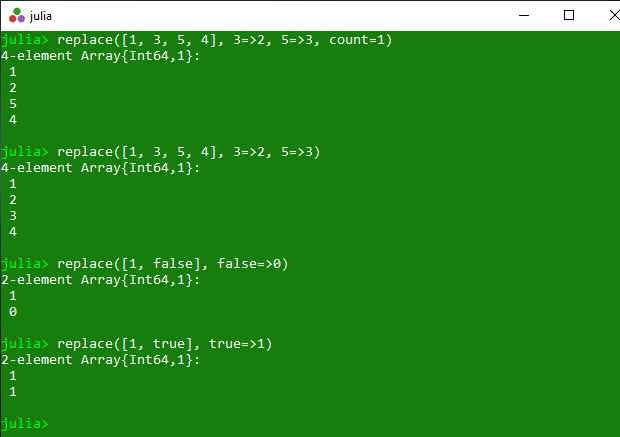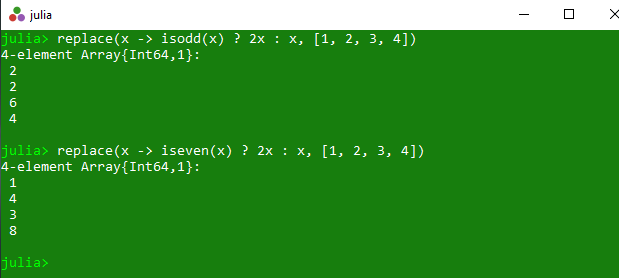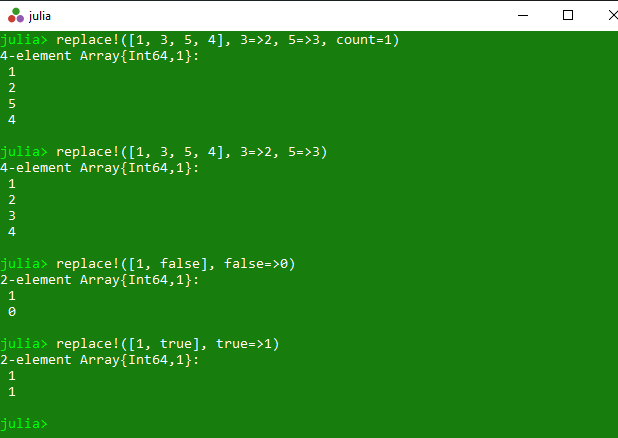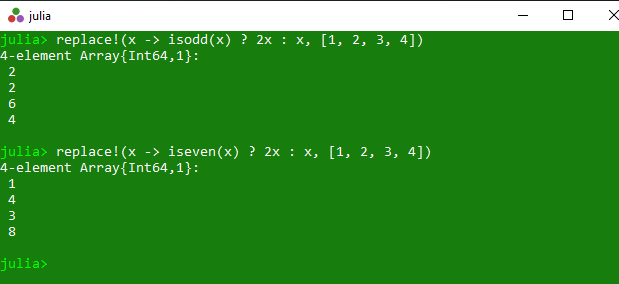# Replacing elements of a collection in Julia – replace() and replace!() Methods

The `replace()` is an inbuilt function in julia which is used to return a copy of the specified array with different types of replacement. These all operations are illustrated with below examples.

Syntax:
replace(A, old_new::Pair…; count::Integer)
or
replace(new::Function, A; count::Integer)

Parameters:

• A: Specified array.
• old_new::Pair: Specified values to get updated.
• count::Integer: This optional count value is used to replace at most count occurrences in total.
• new::Function: Specified set of instruction.

Returns: It returns a copy of the specified array with different types of replacement.

Example 1: This example returns a copy of the specified collection. In this collection old value get replaced with new value and if count is specified, then replace at most count occurrences in total.

 `# Julia program to illustrate  ` `# the use of replace() method ` `  `  `# Getting a copy of the specified collection.  ` `# In this collection old value get replaced  ` `# with new value and if count is specified,  ` `# then replace at most count occurrences in total. ` `println(replace([``1``, ``3``, ``5``, ``4``], ``3` `=``>``2``, ``5` `=``>``3``, count ``=` `1``)) ` `println(replace([``1``, ``3``, ``5``, ``4``], ``3` `=``>``2``, ``5` `=``>``3``)) ` `println(replace([``1``, false], false ``=``>``0``)) ` `println(replace([``1``, true], true ``=``>``1``)) `

Output:Example 2: This example returns a copy of a specified array where each value x in the array is replaced by new(x).

 `# Julia program to illustrate  ` `# the use of replace() method ` `  `  `# Getting a copy of a specified array  ` `# where each value x in the array is  ` `# replaced by new(x). ` `println(replace(x ``-``> isodd(x) ? ``2x` `: x, [``1``, ``2``, ``3``, ``4``])) ` `println(replace(x ``-``> iseven(x) ? ``2x` `: x, [``1``, ``2``, ``3``, ``4``])) `

Output:#### replace!()

The `replace!()` is an inbuilt function in julia which is used to return the replaced specified array with different types of replacement. These all operations are illustrated with below examples.

Syntax:
replace!(A, old_new::Pair…; count::Integer)
or
replace!(new::Function, A; count::Integer)

Parameters:

• A: Specified array.
• old_new::Pair: Specified values to get updated.
• count::Integer: This optional count value is used to replace at most count occurrences in total.
• new::Function: Specified set of instruction.

Returns: It returns the replaced array with different types of replacement method.

Example 1: This example returns the replaced collection. In this collection old value get replaced with new value and if count is specified, then replace at most count occurrences in total.

 `# Julia program to illustrate  ` `# the use of replace !() method ` `  `  `# Getting the replaced collection ` `# In this collection old value get replaced  ` `# with new value and if count is specified,  ` `# then replace at most count occurrences in total. ` `println(replace !([``1``, ``3``, ``5``, ``4``], ``3` `=``>``2``, ``5` `=``>``3``, count ``=` `1``)) ` `println(replace !([``1``, ``3``, ``5``, ``4``], ``3` `=``>``2``, ``5` `=``>``3``)) ` `println(replace !([``1``, false], false ``=``>``0``)) ` `println(replace !([``1``, true], true ``=``>``1``)) `

Output:Example 2: This example returns the replaced specified array where each value x in the array is replaced by new(x).

 `# Julia program to illustrate  ` `# the use of replace !() method ` `  `  `# Getting the replaced specified array  ` `# where each value x in the array is  ` `# replaced by new(x). ` `println(replace !(x ``-``> isodd(x) ? ``2x` `: x, [``1``, ``2``, ``3``, ``4``])) ` `println(replace !(x ``-``> iseven(x) ? ``2x` `: x, [``1``, ``2``, ``3``, ``4``])) `

Output:My Personal Notes arrow_drop_upCheck out this Author's contributed articles.

If you like GeeksforGeeks and would like to contribute, you can also write an article using contribute.geeksforgeeks.org or mail your article to contribute@geeksforgeeks.org. See your article appearing on the GeeksforGeeks main page and help other Geeks.

Please Improve this article if you find anything incorrect by clicking on the "Improve Article" button below.

Article Tags :

Be the First to upvote.

Please write to us at contribute@geeksforgeeks.org to report any issue with the above content.